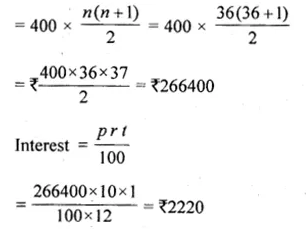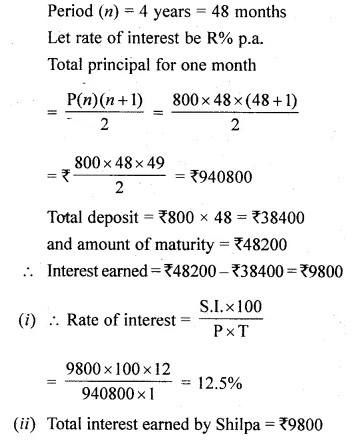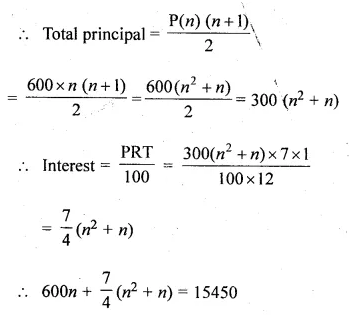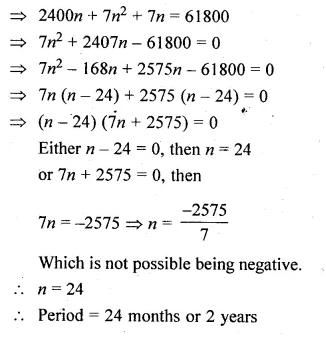## ML Aggarwal Class 10 Solutions for ICSE Maths Chapter 3 Banking Chapter Test

These Solutions are part of ML Aggarwal Class 10 Solutions for ICSE Maths. Here we have given ML Aggarwal Class 10 Solutions for ICSE Maths Chapter 3 Banking Chapter Test

Question 1.
Mr. Dhruv deposits Rs 600 per month in a recurring deposit account for 5 years at the rate of 10% per annum (simple interest). Find the amount he will receive at the time of maturity.
Solution:
Deposit per month = Rs 600
Rate of interest = 10% p.a.
Period (n) = 5 years 60 months.
Total principal for one monthQuestion 2.
Ankita started paying Rs 400 per month in a 3 years recurring deposit. After six months her brother Anshul started paying Rs 500 per month in a $$2 \frac { 1 }{ 2 }$$ years recurring deposit. The bank paid 10% p.a. simple interest for both. At maturity who will get more money and by how much?
Solution:
In case of Ankita,
Deposit per month = Rs 400
Period (n) = 3 years = 36 months
Rate of interest = 10%
Total principal for one monthQuestion 3.
Shilpa has a 4 year recurring deposit account in Bank of Maharashtra and deposits Rs 800 per month. If she gets Rs 48200 at the time of maturity, find
(i) the rate of simple interest,
(ii) the total interest earned by Shilpa
Solution:
Deposit per month (P) = Rs 800
Amount of maturity = Rs 48200Question 4.
Mr. Chaturvedi has a recurring deposit account in Grindlay’s Bank for $$4 \frac { 1 }{ 2 }$$ years at 11% p.a. (simple interest). If he gets Rs 101418.75 at the time of maturity, find the monthly instalment.
Solution:
Let each monthly instalment = Rs x
Rate of interest = 11 %
Period (n) = $$4 \frac { 1 }{ 2 }$$ years or 54 months,
Total principal for one monthQuestion 5.
Rajiv Bhardwaj has a recurring deposit account in a bank of Rs 600 per month. If the bank pays simple interest of 7% p.a. and he gets Rs 15450 as maturity amount, find the total time for which the account was held.
Solution:
Deposit during the month (P) = Rs 600
Rate of interest = 7% p.a.
Amount of maturity = Rs 15450
Let time = n monthsHope given ML Aggarwal Class 10 Solutions for ICSE Maths Chapter 3 Banking Chapter Test are helpful to complete your math homework.

If you have any doubts, please comment below. aplustopper try to provide online math tutoring for you.